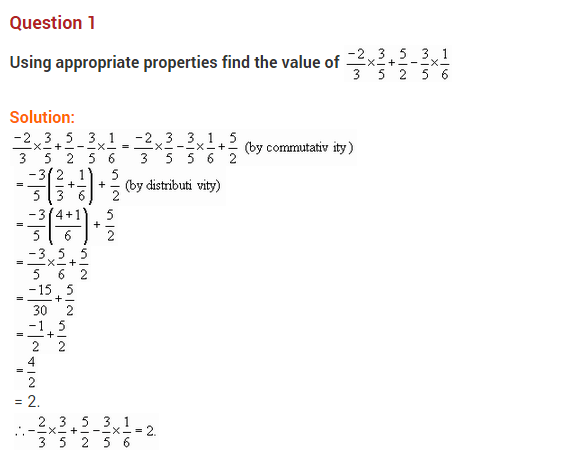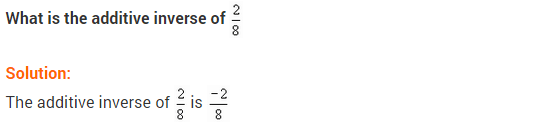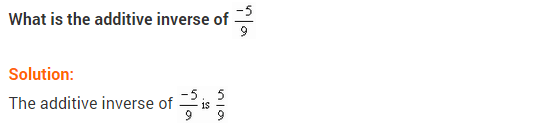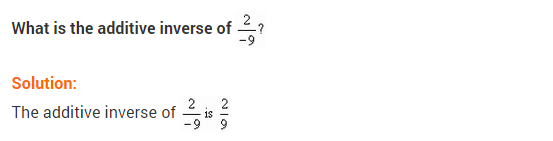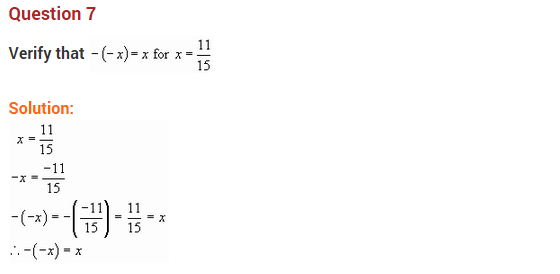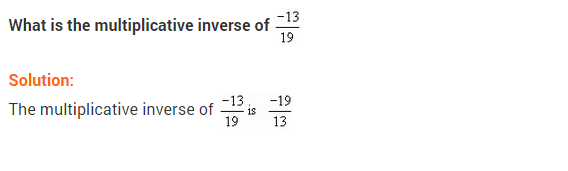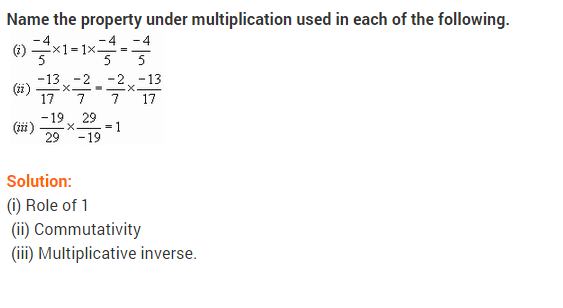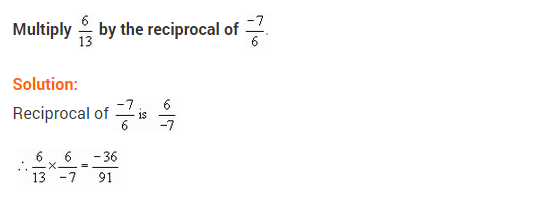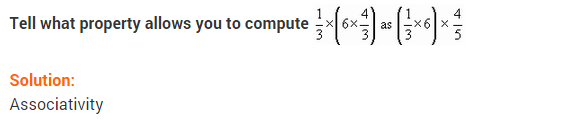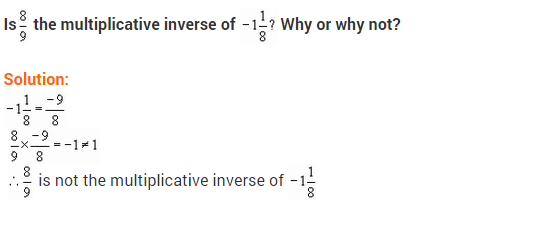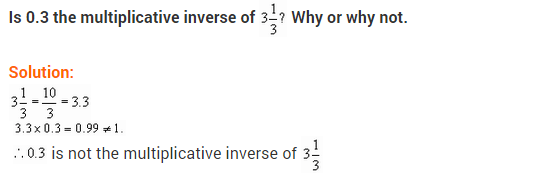## NCERT Solutions for Class 8 Maths Chapter 1 Rational Numbers

NCERT Solutions for Class 8 Maths Chapter 1 Rational Numbers Exercise 1.1

Ex 1.1 Class 8 Maths Question 1.
Using appropriate properties find: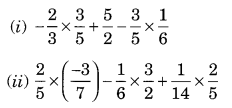Solution: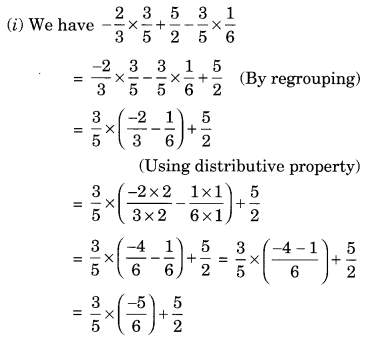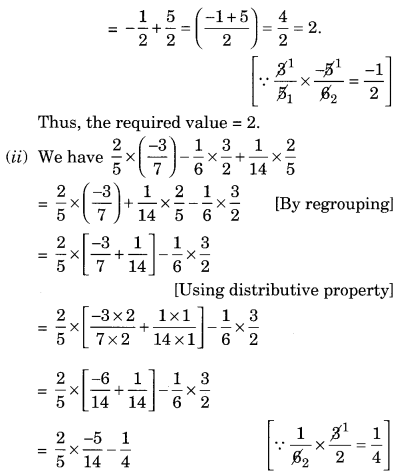Ex 1.1 Class 8 Maths Question 2.
Write the additive inverse of each of the following:
(i) $$\frac { 2 }{ 8 }$$
(ii) $$\frac { -5 }{ 9 }$$
(iii) $$\frac { -6 }{ -5 }$$
(iv) $$\frac { 2 }{ -9 }$$
(v) $$\frac { 19 }{ -6 }$$
Solution: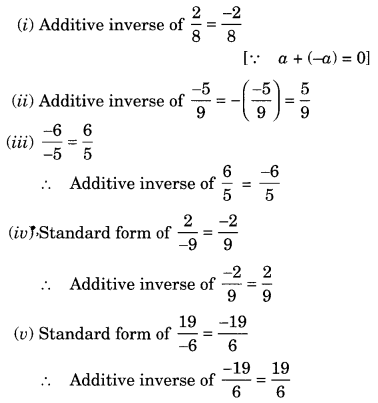Ex 1.1 Class 8 Maths Question 3.
Verify that -(-x) = x for
(i) x = $$\frac { 11 }{ 5 }$$
(ii) x = $$\frac { -13 }{ 17 }$$
Solution: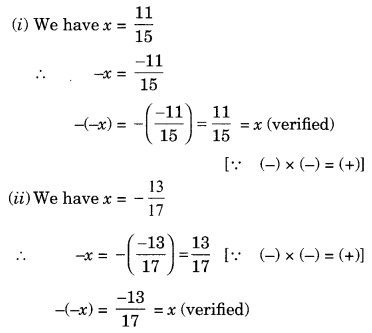Ex 1.1 Class 8 Maths Question 4.
Find the multiplicative inverse of the following:Solution: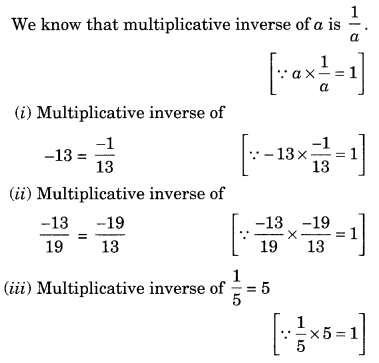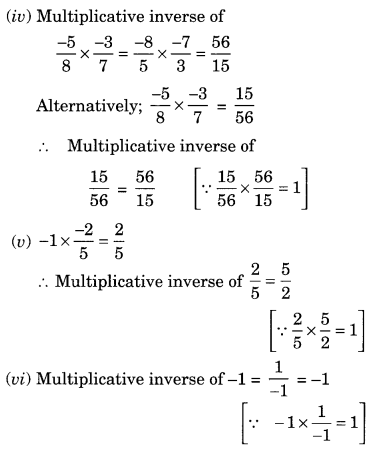Ex 1.1 Class 8 Maths Question 5.
Name the property under multiplication used in each of the following:Solution:
(i) Commutative property of multiplication
(ii) Commutative property of multiplication
(iii) Multiplicative inverse property

Ex 1.1 Class 8 Maths Question 6.
Multiply $$\frac { 6 }{ 13 }$$ by the reciprocal of $$\frac { -7 }{ 16 }$$.
Solution: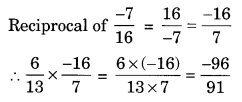Ex 1.1 Class 8 Maths Question 7.
Tell what property allows you to computeSolution:
Since a × (b × c) = (a × b) × c shows the associative property of multiplications.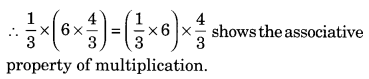Ex 1.1 Class 8 Maths Question 8.
Is $$\frac { 8 }{ 9 }$$ the multiplicative inverse of -1$$\frac { 1 }{ 8 }$$? Why or Why not?
Solution:
Here -1$$\frac { 1 }{ 8 }$$ = $$\frac { -9 }{ 8 }$$.
Since multiplicative inverse of $$\frac { 8 }{ 9 }$$ is $$\frac { 9 }{ 8 }$$ but not $$\frac { -9 }{ 8 }$$
$$\frac { 8 }{ 9 }$$ is not the multiplicative inverse of -1$$\frac { 1 }{ 8 }$$

Ex 1.1 Class 8 Maths Question 9.
If 0.3 the multiplicative inverse of 3$$\frac { 1 }{ 3 }$$? Why or why not?
Solution:Multiplicative inverse of 0.3 or $$\frac { 3 }{ 10 }$$ is $$\frac { 10 }{ 3 }$$.
Thus, 0.3 is the multiplicative inverse of 3$$\frac { 1 }{ 3 }$$.

Ex 1.1 Class 8 Maths Question 10.
Write:
(i) The rational number that does not have a reciprocal.
(ii) The rational numbers that are equal to their reciprocals.
(iii) The rational number that is equal to its negative.
Solution:
(i) 0 is the rational number which does not have its reciprocal
[∵ $$\frac { 1 }{ 0 }$$ is not defined]
(ii) Reciprocal of 1 = $$\frac { 1 }{ 1 }$$ = 1
Reciprocal of -1 = $$\frac { 1 }{ -1 }$$ = -1
Thus, 1 and -1 are the required rational numbers.
(iii) 0 is the rational number which is equal to its negative.

Ex 1.1 Class 8 Maths Question 11.
Fill in the blanks.
(i) Zero has ……….. reciprocal.
(ii) The numbers ……….. and ……….. are their own reciprocals.
(iii) The reciprocal of -5 is ………
(iv) Reciprocal of $$\frac { 1 }{ x }$$, where x ≠ 0 is ……….
(v) The product of two rational numbers is always a …………
(vi) The reciprocal of a positive rational number is ……….
Solution:
(i) no
(ii) -1 and 1
(iii) $$\frac { -1 }{ 5 }$$
(iv) x
(v) rational number
(vi) positive